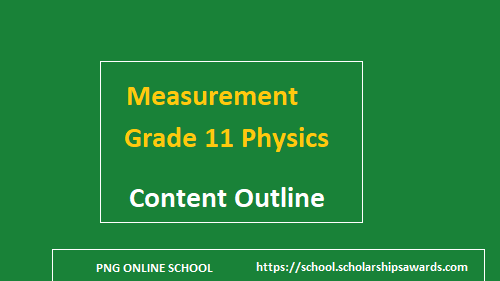# Measurement Grade 11 Physics Unit

Measurement is one of the units   in  the Grade 11 Physics  content   taught in PNG in both the PNG Standard Based school Curriculum & STEM curriculums and is basically assigning numerical values to quantities with defined units which may be used for comparison. The physical quantities measured can be used to describe its fundamental nature.Measurement Grade 11 Physics Unit

Measurement introduces the student into effectively demonstrating the use of basic scientific instruments and analyse the readings to a reasonable degree of accuracy. This module provides students with an understanding of measurement including quantities, units and error analysis. Students can use this skill and knowledge to solve similar measurements related problems in other subjects and in the real world.

Measurement is Unit 1 on the  five units in the Grade 11 physics subjects. In Grade 11, the units covered in PNG Secondary Schools and National High Schools are.

• 11.1  Measurement
• 11. 2. Motion (Kinematics)
• 11.3 Force and Motion
• 11.4  Work, Power and Energy
• 11.5 Electrical Principles
• 11.6 Electronics
The following content must be taught and learned in the Grade 11 Physics unit 11.1 Measurement
Content Benchmark

Student must master knowledge and skills of;
1. Quantities and units
2. Measuring Instruments
3. Dimensional Analysis
4. Error Analysis
5. Graphs

#### Quantities and Units in Measurement

Students must master the following content under this topic.
•  Define measurements
• Identify basic quantities and derived quantities
• Classify physical quantities as scalar and vectors
•  Use and Convert Internationally accepted units of measurement and prefixes
• Arrange order of magnitude of quantities
• Converting numbers into scientific notation and identifying number of significant figures.

#### Measuring Instruments

Under this topic, students must master the following content.
• Apply correct techniques to get readings using measuring instruments
• Carry out simple experiments using measuring instruments

#### Dimensional Analysis

Under this topic, students must learn the following content
• Define Dimensional Analysis
• State the three commonly used fundamental units applied in dimensional analysis.
• Perform dimensional analysis to examine the validity of a formula.

#### Error Analysis

Under the topic Error analysis, students must learn the following content
•  Define error
• Differentiate the different types of errors
• Solve related problems

#### Graphs in Measurement

Students must learn the following content under this topic.

• Define dependent and independent variable
• Draw a graph by plotting points on a Cartesian axis
• Estimate, predict and interpret data from a graph

Also check out